This function calculates explanations on a dataset level set that explore model response as a function of selected variables. The explanations can be calulated as Partial Dependence Profile or Accumulated Local Dependence Profile. Find information how to use this function here: https://ema.drwhy.ai/partialDependenceProfiles.html. The variable_profile function is a copy of model_profile.

model_profile(
explainer,
variables = NULL,
N = 100,
...,
groups = NULL,
k = NULL,
center = TRUE,
type = "partial"
)

variable_profile(
explainer,
variables = NULL,
N = 100,
...,
groups = NULL,
k = NULL,
center = TRUE,
type = "partial"
)

single_variable(explainer, variable, type = "pdp", ...)

## Arguments

explainer

a model to be explained, preprocessed by the explain function

variables

character - names of variables to be explained

N

number of observations used for calculation of aggregated profiles. By default 100. Use NULL to use all observations.

...

other parameters that will be passed to ingredients::aggregate_profiles

groups

a variable name that will be used for grouping. By default NULL which means that no groups shall be calculated

k

number of clusters for the hclust function (for clustered profiles)

center

shall profiles be centered before clustering

type

the type of variable profile. Either partial, conditional or accumulated.

variable

## Value

An object of the class model_profile. It's a data frame with calculated average model responses.

## Details

Underneath this function calls the partial_dependence or accumulated_dependence functions from the ingredients package.

## Examples

titanic_glm_model <- glm(survived~., data = titanic_imputed, family = "binomial")
explainer_glm <- explain(titanic_glm_model, data = titanic_imputed)
#> Preparation of a new explainer is initiated
#>   -> model label       :  lm  (  default  )
#>   -> data              :  2207  rows  8  cols
#>   -> target variable   :  not specified! (  WARNING  )
#>   -> predict function  :  yhat.glm  will be used (  default  )
#>   -> predicted values  :  No value for predict function target column. (  default  )
#>   -> model_info        :  package stats , ver. 4.2.3 , task classification (  default  )
#>   -> model_info        :  Model info detected classification task but 'y' is a NULL .  (  WARNING  )
#>   -> model_info        :  By deafult classification tasks supports only numercical 'y' parameter.
#>   -> model_info        :  Consider changing to numerical vector with 0 and 1 values.
#>   -> model_info        :  Otherwise I will not be able to calculate residuals or loss function.
#>   -> predicted values  :  numerical, min =  0.008128381 , mean =  0.3221568 , max =  0.9731431
#>   -> residual function :  difference between y and yhat (  default  )
#>   A new explainer has been created!
model_profile_glm_fare <- model_profile(explainer_glm, "fare")
plot(model_profile_glm_fare)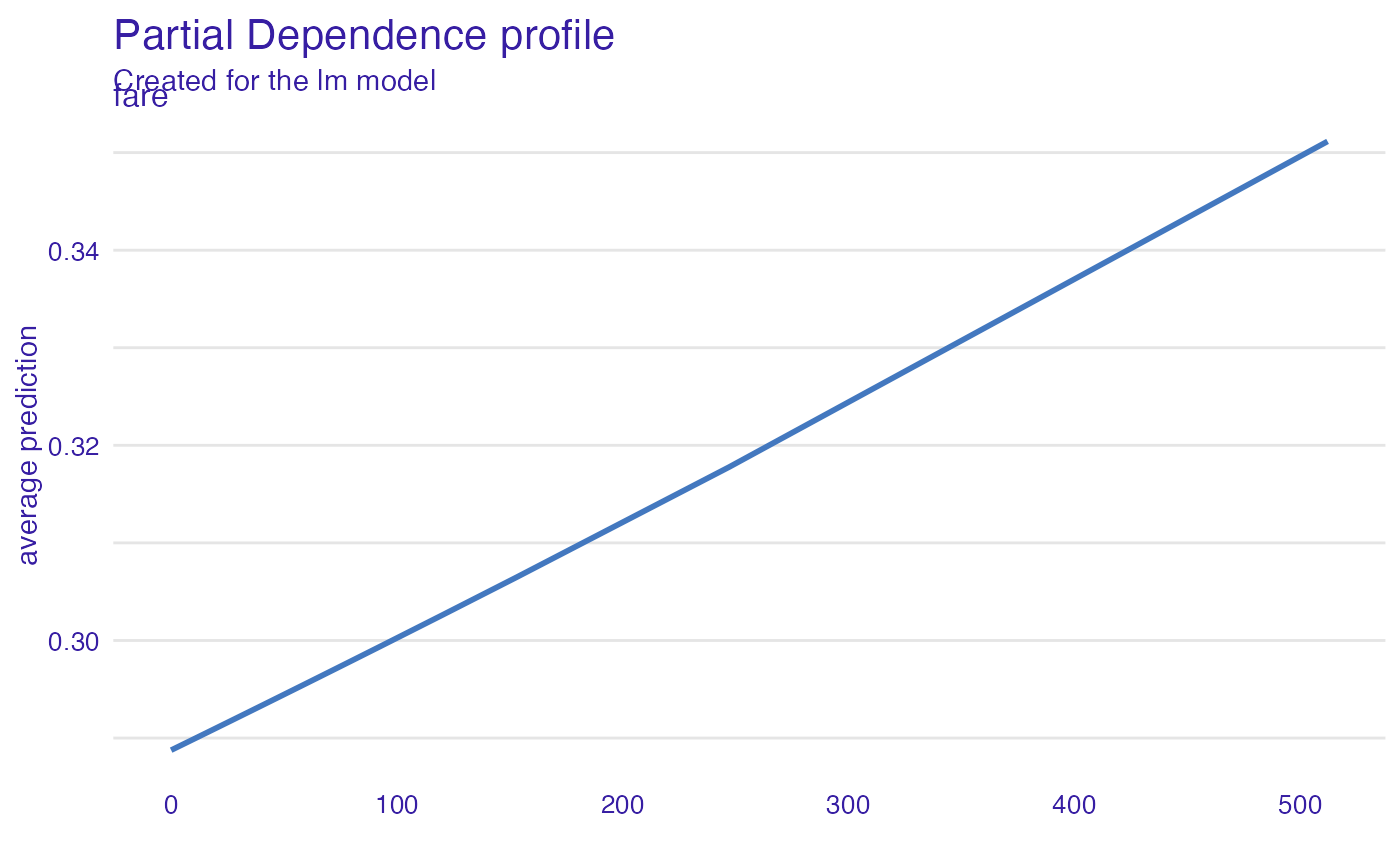# \donttest{
library("ranger")
titanic_ranger_model <- ranger(survived~., data = titanic_imputed, num.trees = 50,
probability = TRUE)
explainer_ranger  <- explain(titanic_ranger_model, data = titanic_imputed)
#> Preparation of a new explainer is initiated
#>   -> model label       :  ranger  (  default  )
#>   -> data              :  2207  rows  8  cols
#>   -> target variable   :  not specified! (  WARNING  )
#>   -> predict function  :  yhat.ranger  will be used (  default  )
#>   -> predicted values  :  No value for predict function target column. (  default  )
#>   -> model_info        :  package ranger , ver. 0.14.1 , task classification (  default  )
#>   -> model_info        :  Model info detected classification task but 'y' is a NULL .  (  WARNING  )
#>   -> model_info        :  By deafult classification tasks supports only numercical 'y' parameter.
#>   -> model_info        :  Consider changing to numerical vector with 0 and 1 values.
#>   -> model_info        :  Otherwise I will not be able to calculate residuals or loss function.
#>   -> predicted values  :  numerical, min =  0.007194627 , mean =  0.3204426 , max =  0.9986442
#>   -> residual function :  difference between y and yhat (  default  )
#>   A new explainer has been created!
model_profile_ranger <- model_profile(explainer_ranger)
plot(model_profile_ranger, geom = "profiles")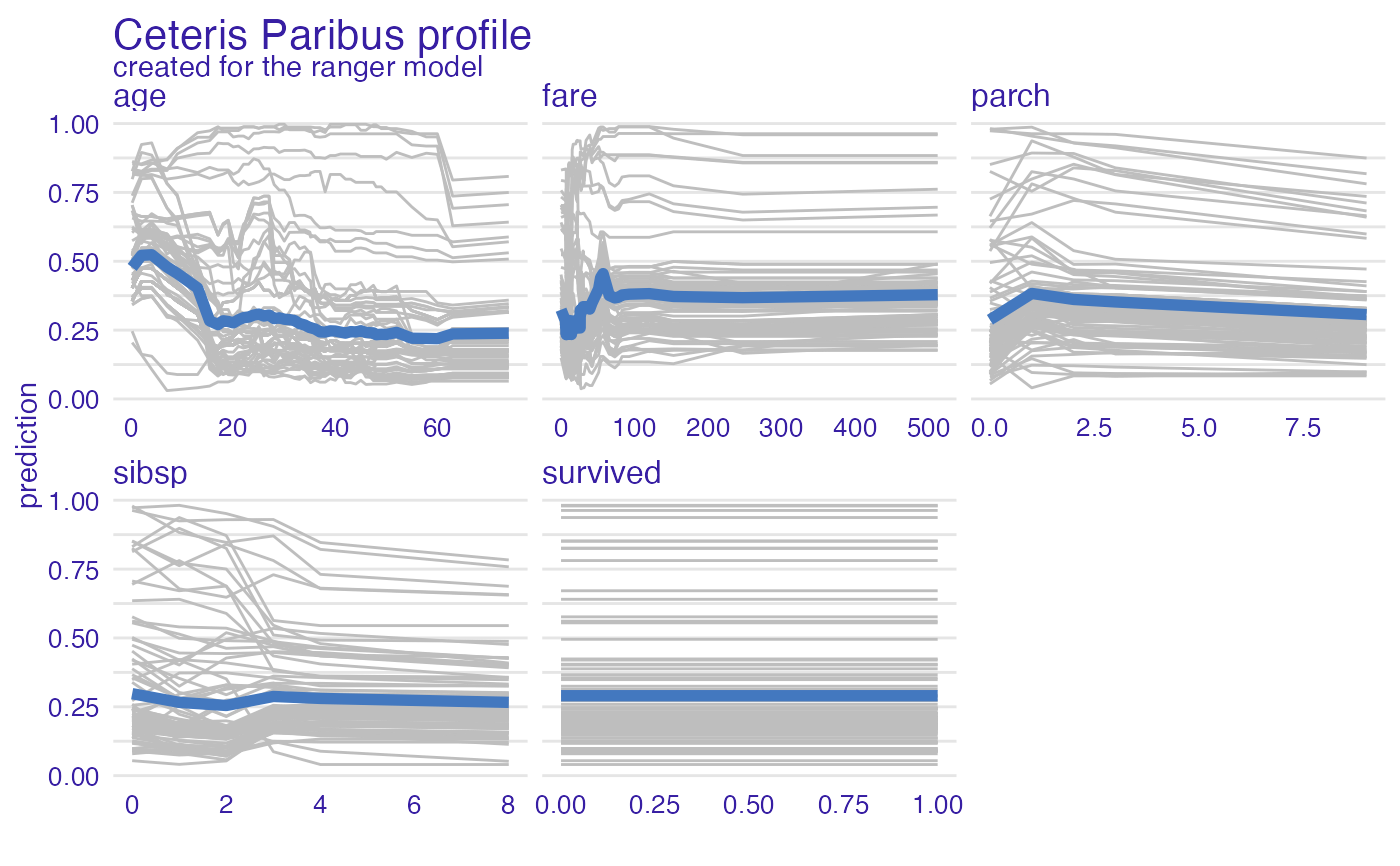model_profile_ranger_1 <- model_profile(explainer_ranger, type = "partial",
variables = c("age", "fare"))
plot(model_profile_ranger_1 , variables = c("age", "fare"), geom = "points")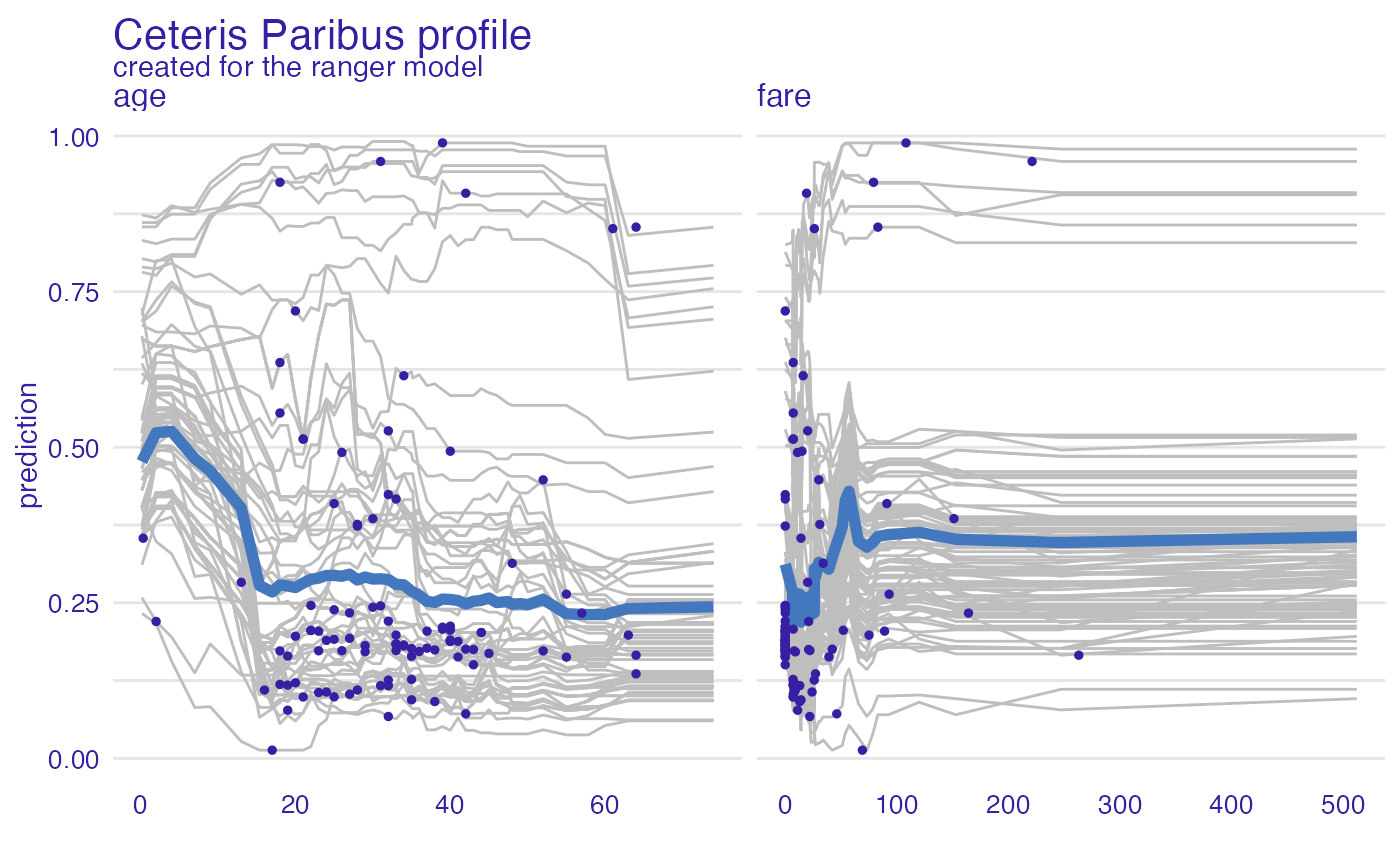model_profile_ranger_2  <- model_profile(explainer_ranger, type = "partial", k = 3)
plot(model_profile_ranger_2 , geom = "profiles")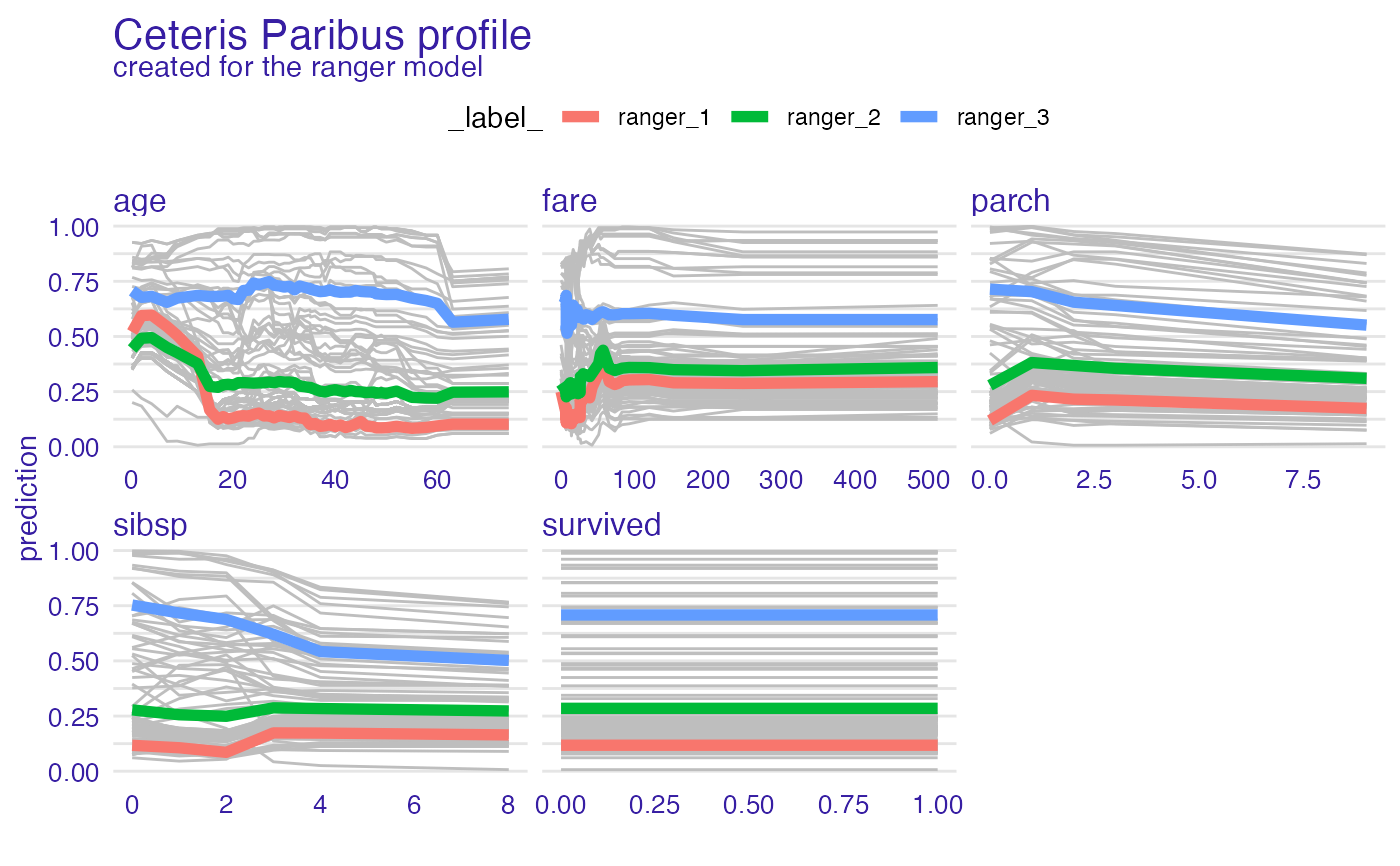model_profile_ranger_3  <- model_profile(explainer_ranger, type = "partial", groups = "gender")
plot(model_profile_ranger_3 , geom = "profiles")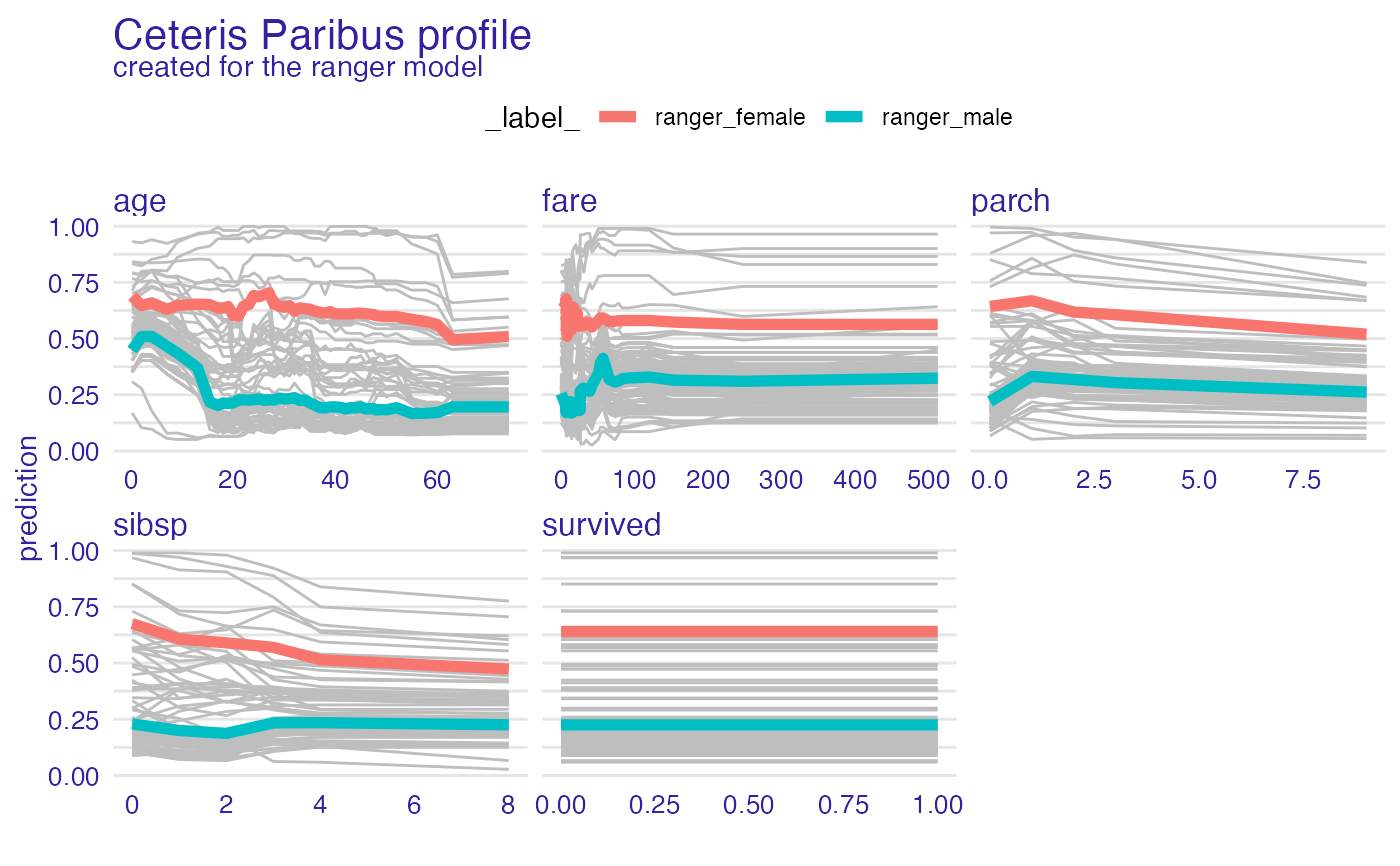model_profile_ranger_4  <- model_profile(explainer_ranger, type = "accumulated")
plot(model_profile_ranger_4 , geom = "profiles")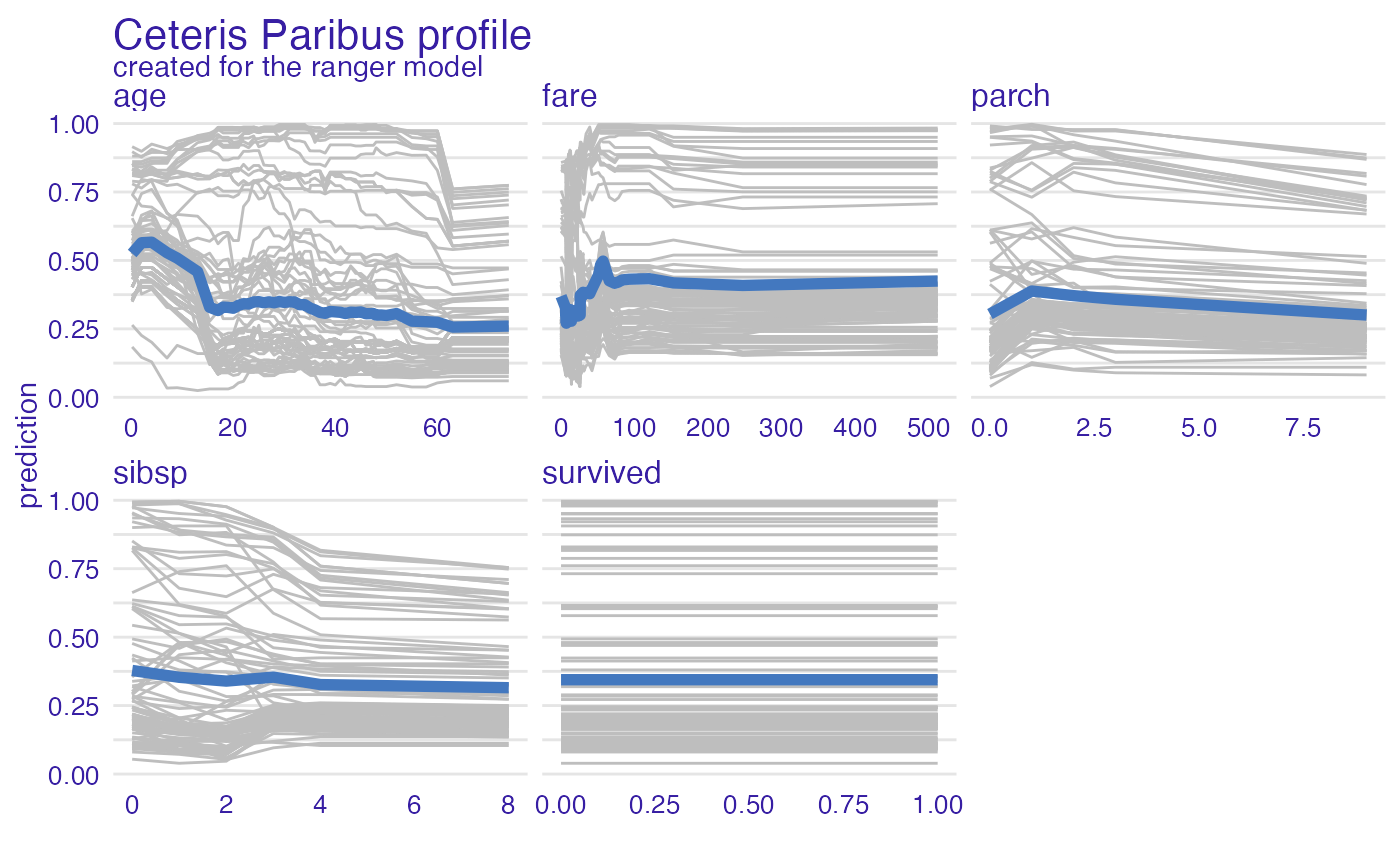# Multiple profiles
model_profile_ranger_fare <- model_profile(explainer_ranger, "fare")
plot(model_profile_ranger_fare, model_profile_glm_fare)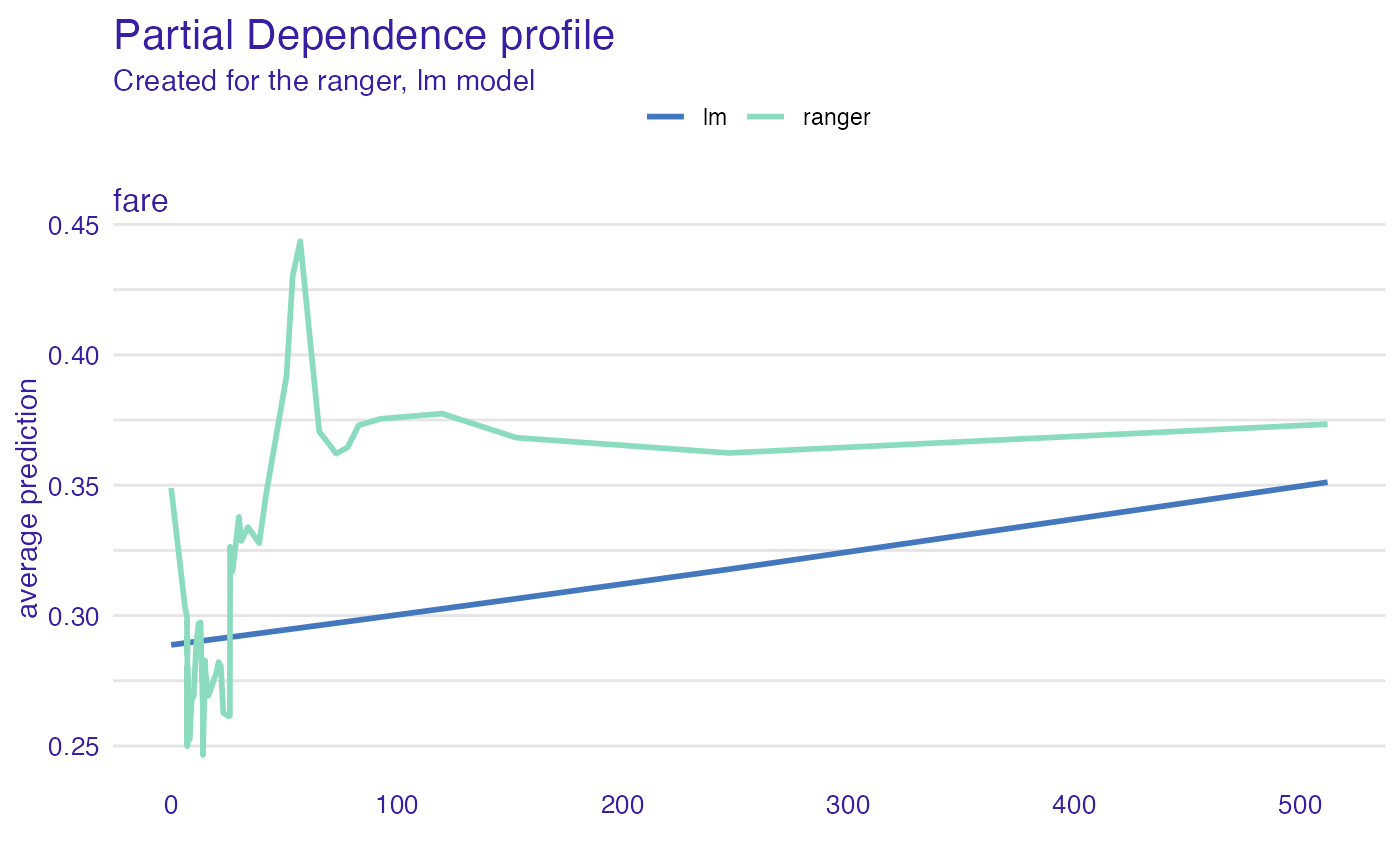# }